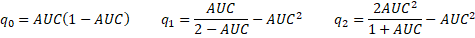# AUC Confidence Interval

For large samples, AUC (area under the curve for a ROC curve) is approximately normally distributed, and so a 1-α confidence interval for AUC may be calculated as described in Confidence Interval for Sampling Distributions.

The confidence interval is equal to AUC  ± se · zcrit where zcrit is the two-tailed critical value of the standard normal distribution, as calculated in Excel by =NORM.S.INV(1-α/2) andwhere n1 and n2 are the sizes of the two samples andExample 1: Find the 95% confidence for the AUC from Example 1 of Classification Table.

From Figure 1 of ROC Curve, we see that n1 = 527, n2 = 279 and AUC = .88915. The 95% confidence interval of AUC is (.86736, .91094), as shown in Figure 1.Figure 1 – AUC 95% confidence Interval

Real Statistics Functions: The Real Statistics Resource Pack contains the following functions:

AUC_LOWER(aucn1, n2, α) = the lower limit of the 1-α  confidence interval for the area under the curve = auc for samples of size n1 and n2

AUC_UPPER(aucn1, n2, α) = the upper limit of the 1-α confidence interval for the area under the curve = auc for samples of size n1 and n2

If the α argument is omitted it defaults to .05.

For Example 1, we see that =AUC_LOWER(B5, B3, B4) calculates the value shown in cell B12 and =AUC_UPPER(B5, B3, B4) calculates the value shown in cell B13.

# Personal Career & Learning Guide for Data Analyst, Data Engineer and Data Scientist

## Applied Machine Learning & Data Science Projects and Coding Recipes for Beginners

A list of FREE programming examples together with eTutorials & eBooks @ SETScholars

# Projects and Coding Recipes, eTutorials and eBooks: The best All-in-One resources for Data Analyst, Data Scientist, Machine Learning Engineer and Software Developer

Topics included: Classification, Clustering, Regression, Forecasting, Algorithms, Data Structures, Data Analytics & Data Science, Deep Learning, Machine Learning, Programming Languages and Software Tools & Packages.
(Discount is valid for limited time only)`Disclaimer: The information and code presented within this recipe/tutorial is only for educational and coaching purposes for beginners and developers. Anyone can practice and apply the recipe/tutorial presented here, but the reader is taking full responsibility for his/her actions. The author (content curator) of this recipe (code / program) has made every effort to ensure the accuracy of the information was correct at time of publication. The author (content curator) does not assume and hereby disclaims any liability to any party for any loss, damage, or disruption caused by errors or omissions, whether such errors or omissions result from accident, negligence, or any other cause. The information presented here could also be found in public knowledge domains.`

# Learn by Coding: v-Tutorials on Applied Machine Learning and Data Science for Beginners

Please do not waste your valuable time by watching videos, rather use end-to-end (Python and R) recipes from Professional Data Scientists to practice coding, and land the most demandable jobs in the fields of Predictive analytics & AI (Machine Learning and Data Science).

The objective is to guide the developers & analysts to “Learn how to Code” for Applied AI using end-to-end coding solutions, and unlock the world of opportunities!